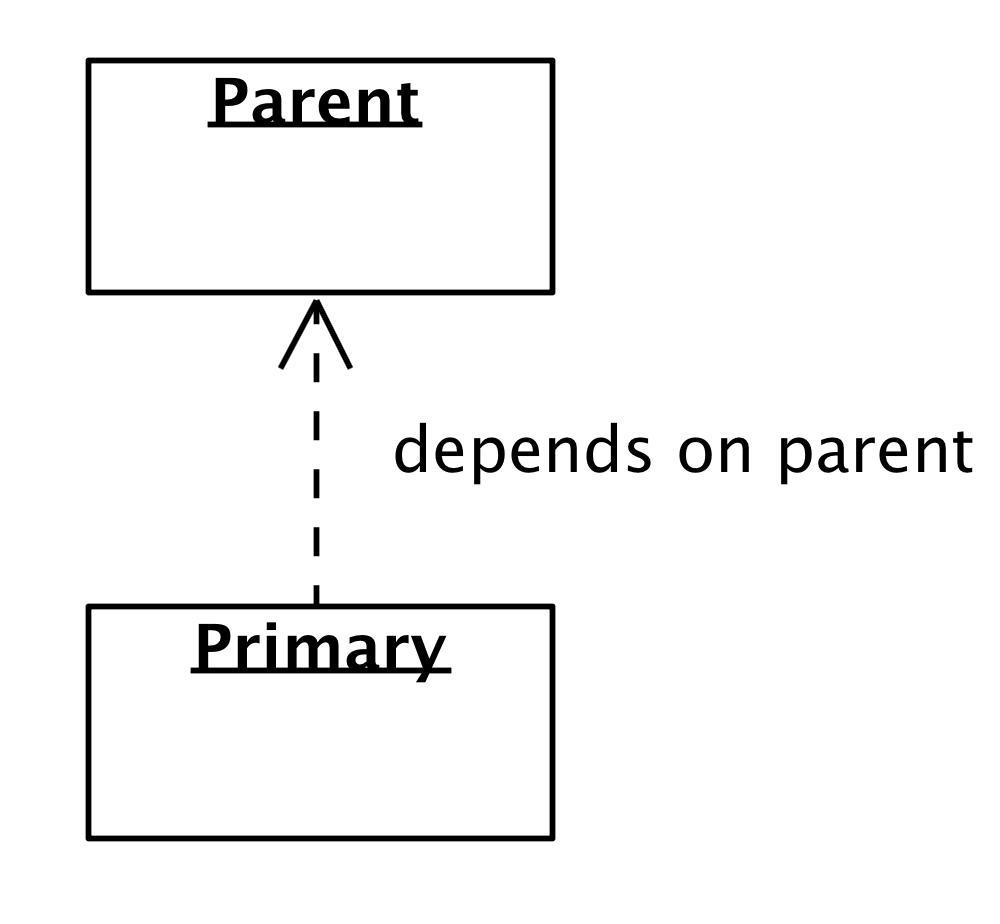# Definition

The Primary Domain test data generation is dependent on the Parent Domain test data generation. Each time the Parent Domain completes an iteration of test data generation, the Primary Domain is called to complete one or more iterations of its test data generation with respect to the Parent Domain.

## Object Diagram## Parent-Primary Patterns

The Primary Domain can have three possible test data generation patterns with respect to the Parent Domain:

1. One to One (1-1) Parent-Primary Pattern: For each generated record of the Parent Domain, generate exactly one record for the Primary Domain.

2. Fixed One to Many (1-N) Parent-Primary Pattern: For each generated record of the Parent Domain, generate exactly N records for the Primary Domain where N is a constant greater than 1.

3. Dynamic Zero to Many (0-M) Parent-Primary Pattern: For each generated record of the Parent Domain, generate M records for the Primary Domain where M is a randomly generated number between 0 and N.

# Parent-Primary Pattern Iteration Examples

## Pseudo Code Examples

The Pseudo code below shows the sequence in which test data is generated for the different Parent-Primary patterns.

### One to One (1-1) Parent-Primary Pattern

```for (parentLoop in 1..N) do
parent.generateNext()
for (primaryLoop in 1..1) do
primary.generateNext()```

### Fixed One to Many (1-N) Parent-Primary Pattern

```for (parentLoop in 1..N) do
parent.generateNext()
for (primaryLoop in 1..M) do
primary.generateNext()```

### Dynamic Zero to Many (0-M) Parent-Primary Pattern

```for (parentLoop in 1..N) do
parent.generateNext()
M = randomNumber(0..X)
while (--M > 0) do
primary.generateNext()```

## Concrete Examples

The following are concrete examples where the Parent Domain is a Customer Domain and the Primary Domain is an Address Domain.

### One to One (1-1) Parent-Primary Pattern

For each Customer, generate one and only one Address.

Example:
•    If number of Customers = 1000
•    Then number of generated Addresses = 1000

### Fixed One to Many (1-N) Parent-Primary Pattern

For each Customer, generate exactly N Addresses.

Example:
•    If number of Customers = 1000
•    N = 3
•    Then number of generated Addresses = 3000

### Dynamic Zero to Many (0-M) Parent-Primary Pattern

For each Customer, randomly generate between 0 and 5 Addresses.

Example:
•    If number of Customers = 1000
•    N = random number between 0 and 5
•    Each customer may have 0 to 5 Addresses
•    Then the average number of generated Addresses = 2500